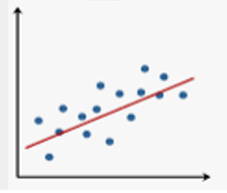Mathematics
Easy

Question

# What type of association is in this scatter plot?## Linear association  Nonlinear association No correlation Negative associationHint:

## The correct answer is: Linear association

### The given picture is in the linear association.step 1 . A scatter plot shows the relation between two variables. It uses dots (.)  to show the relation between two variable .Step 2. In the given diagram we can clearly see that the points areConclusion ;By the definition of linear association 'we can say that the above scatter plot diagram shows linear association

step 1 . A scatter plot shows the relation between two variables. It uses dots (.)  to show the relation between two variable .
Step 2. In the given diagram we can clearly see that the points are
Conclusion ;
By the definition of linear association 'we can say that the above scatter plot diagram shows linear association

### Related Questions to study#### With Turito Foundation.#### Get an Expert Advice From Turito.